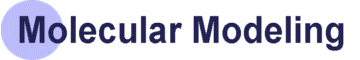Computational Methods Computational Methods are different mathematical means of calculating the potential energy surface of a molecule. The potential energy surface of a molecule is the accumulation of all the forces due to interacton between atoms of a molecule. The Computational Methods can be divided into two major categories based on the chemical theories that they are derived from: Molecular Mechanical Methods and Quantum Mechanical Methods. Quantum Mechanical Methods include two basic types: Semi-Emperical and ab initio. Molecular Mechanical Methods Quantum Mechanical Methods: Semi-Empirical ab initio The potential energy surface can be used to determine theoretical and physical properties of a molecule. These properties include everything from coordinate positions of atoms to heats of formation. The uses of computational methods can be divided into 3 categories: Single Point Energy, Geometry Optimization, and Property Calculations. Single Point Energy Geometry Optimization Property Calculation